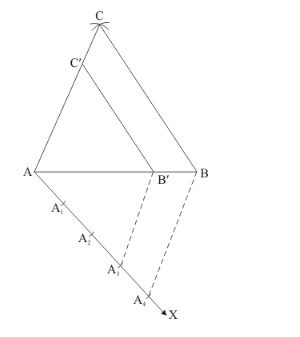# Draw a ΔABC in which BC = 6 cm, AB = 4 cm and AC = 5 cm.

Question:

Draw a ΔABC in which BC = 6 cm, AB = 4 cm and AC = 5 cm. Draw a triangle similar to ΔABC with its sides equal to (3/4)th of the corresponding sides of ΔABC.

Solution:

Given that

Construct a triangle of sides $A B=4 \mathrm{~cm}, B C=6 \mathrm{~cm}$ and $A C=5 \mathrm{~cm}$ and then a triangle similar to it whose sides are $(3 / 4)^{\text {th }}$ of the corresponding sides of $\triangle A B C$.

We follow the following steps to construct the givenStep of construction

Step: I- First of all we draw a line segment.

Step: II- With as centre and radius, draw an arc.

Step: III -With as centre and radius, draw an arc, intersecting the arc drawn in step II at C.

Step: IV -Joins AC and BC to obtain.

Step: $V$-Below $A B$, makes an acute angle $\angle B A X=60^{\circ}$.

Step: VI -Along $A X$, mark off four points $A_{1}, A_{2} A_{3}$ and $A_{4}$ such that $A A_{1}=A_{1} A_{2}=A_{2} A_{3}=A_{3} A_{4}$

Step: VII $-J \operatorname{Join} A_{4} B$

Step: VIII - Since we have to construct a triangle each of whose sides is $(3 / 4)^{\text {th }}$ of the corresponding sides of $\triangle A B C$.

So, we take three parts out of four equal parts on $A X$ from point $A_{3}$ draw $A_{3} B^{\prime} \| A_{4} B$, and meeting $A B$ at $B$ '.

Step: IX- From $B^{\prime}$ draw $B^{\prime} C \| B C$, and meeting $A C$ at $C^{\prime}$

Thus, $\triangle A B^{\prime} C^{\prime}$ is the required triangle, each of whose sides is $(3 / 4)^{\text {th }}$ of the corresponding sides of $\triangle A B C$.